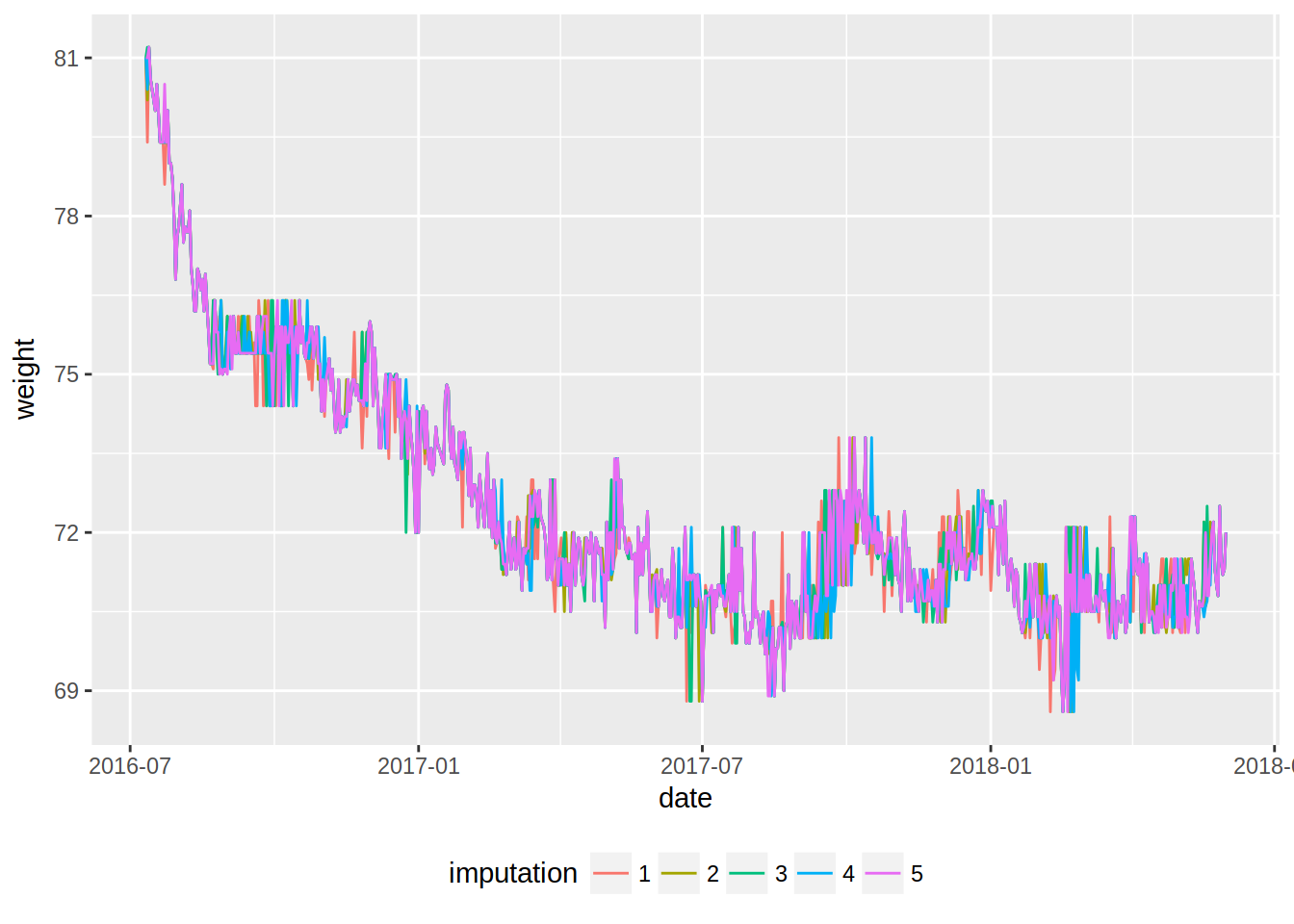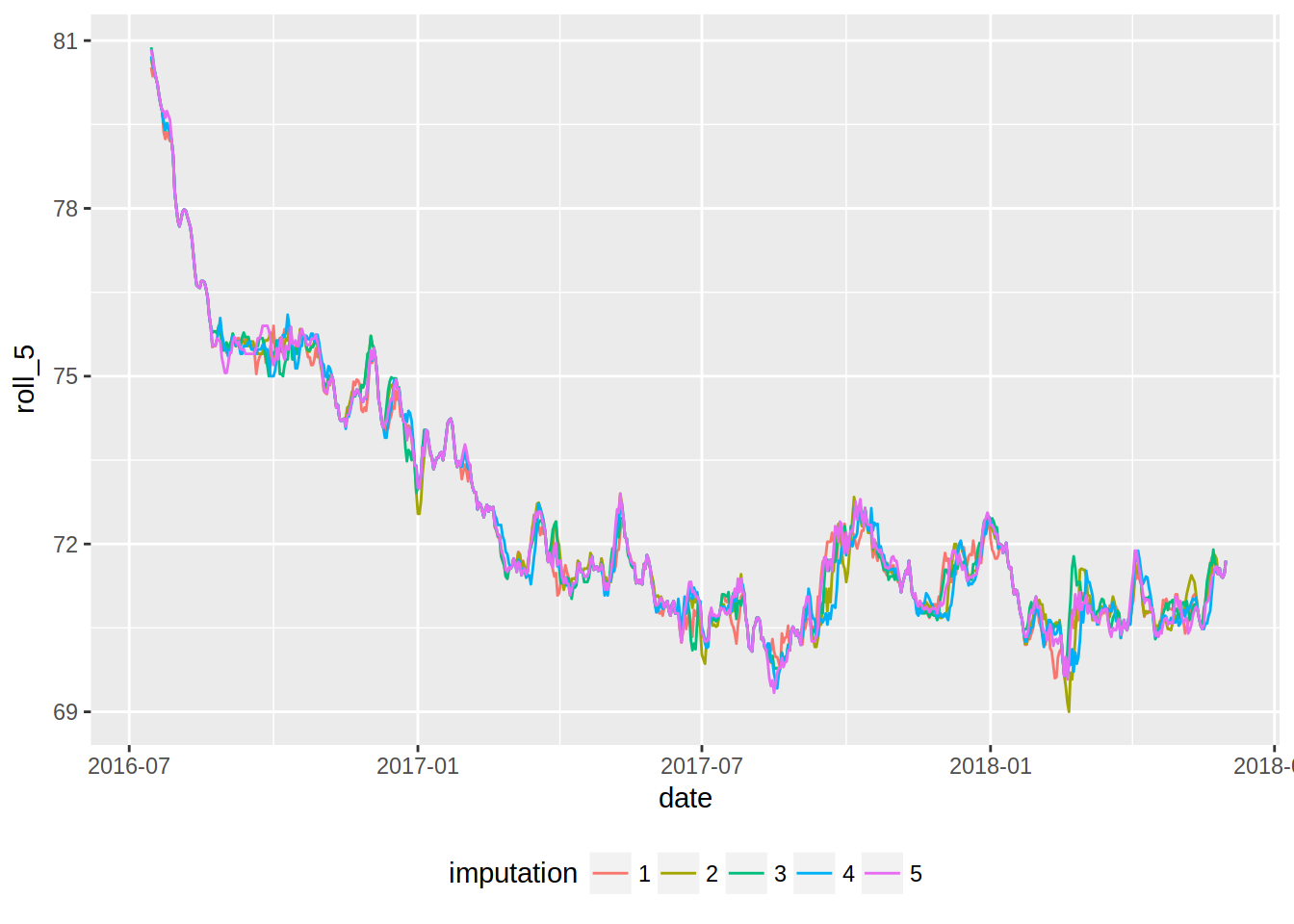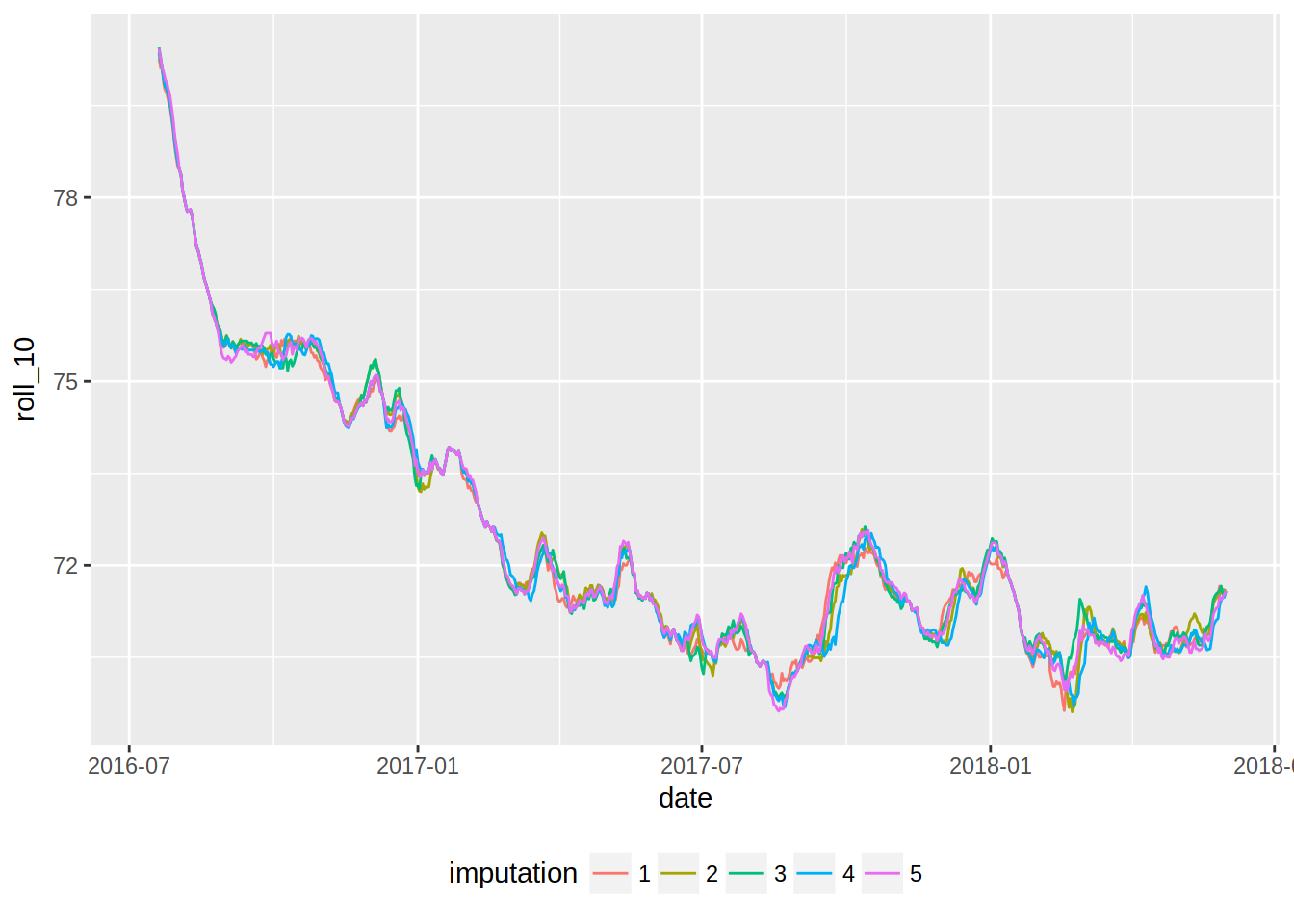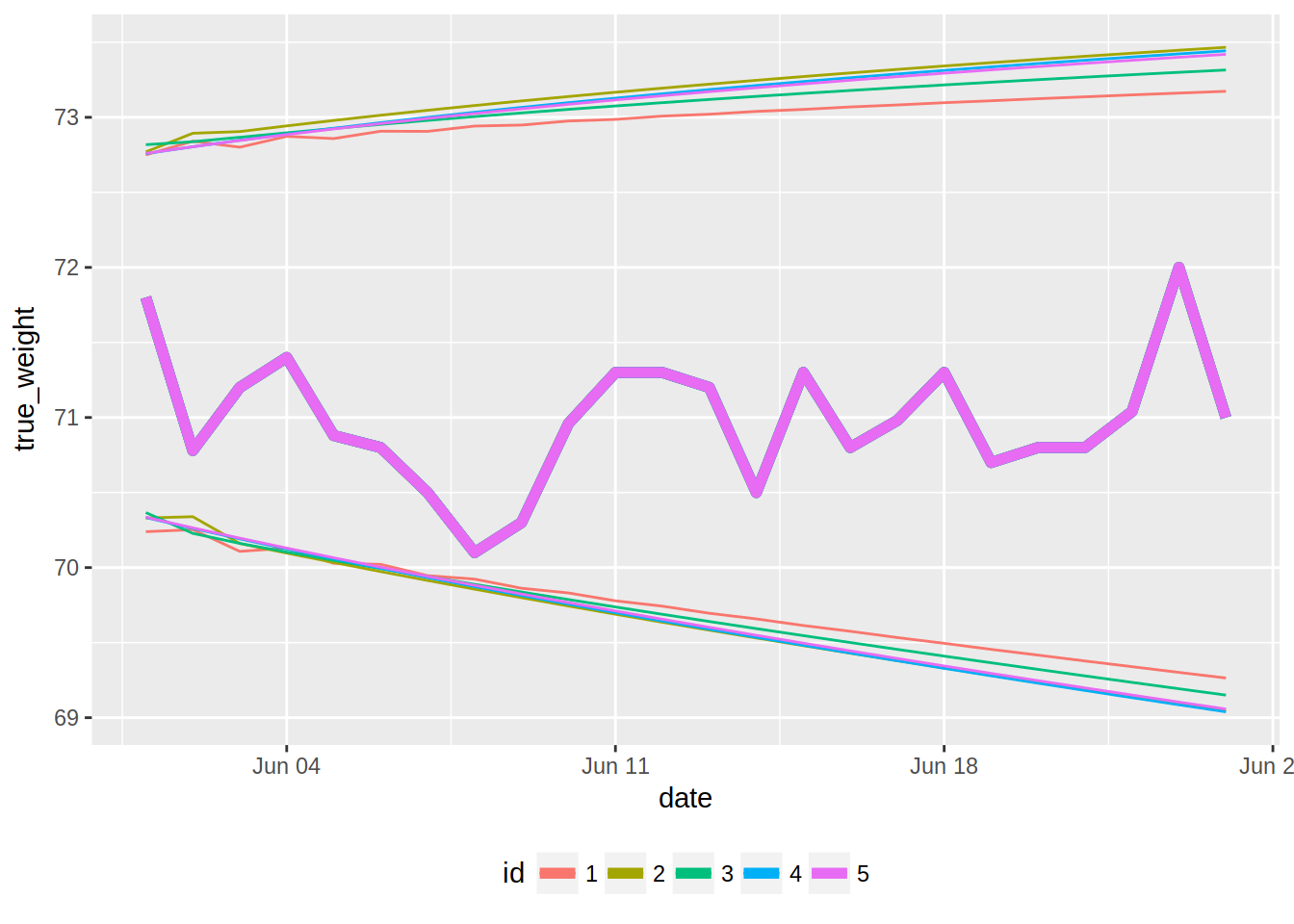```Econometrics and Free Software by Bruno Rodrigues.
Check out my package that adds logging to R functions, {chronicler}.
Or read my free ebooks, to learn some R and build reproducible analytical pipelines..
You can also watch my youtube channel or find the slides to the talks I've given here.
Buy me a coffee, my kids don't let me sleep.
```

# Forecasting my weight with R

R

I’ve been measuring my weight almost daily for almost 2 years now; I actually started earlier, but not as consistently. The goal of this blog post is to get re-acquaiented with time series; I haven’t had the opportunity to work with time series for a long time now and I have seen that quite a few packages that deal with time series have been released on CRAN. In this blog post, I will explore my weight measurements using some functions from the `{tsibble}` and `{tibbletime}` packages, and then do some predictions with the `{forecast}` package.

First, let’s load the needed packages, read in the data and convert it to a `tsibble`:

``````library("tidyverse")
library("forecast")
library("tsibble")
library("tibbletime")
library("mice")``````
``````weight <- read_csv("https://gist.githubusercontent.com/b-rodrigues/ea60679135f8dbed448ccf66a216811f/raw/18b469f3b0720f76ce5ee2715d0f9574b615f170/gistfile1.txt") %>%
as_tsibble()``````
``````## Parsed with column specification:
## cols(
##   Date = col_date(format = ""),
##   Poids = col_double()
## )``````
``## The `index` is `Date`.``

You can read more about `{tsibble}` here. Here, I use `{tsibble}` mostly for the next step, which is using the function `fill_na()` on the tsibble. `fill_na()` turns implicit missing values into explicit missing values. These are implicit missing values:

``````          Date Poids
1   2013-01-01 84.10
2   2013-01-04 85.60``````

and this is the same view, but with explicit missing values:

``````          Date Poids
1   2013-01-01 84.10
2   2013-01-02 NA
3   2013-01-03 NA
4   2013-01-04 85.60``````

This is useful to do, because I want to impute the missing values using the `{mice}` package. Let’s do this:

``````weight <- weight %>%
fill_na()

imp_weight <- mice(data = weight) %>%
mice::complete("long")``````
``````##
##  iter imp variable
##   1   1  Poids
##   1   2  Poids
##   1   3  Poids
##   1   4  Poids
##   1   5  Poids
##   2   1  Poids
##   2   2  Poids
##   2   3  Poids
##   2   4  Poids
##   2   5  Poids
##   3   1  Poids
##   3   2  Poids
##   3   3  Poids
##   3   4  Poids
##   3   5  Poids
##   4   1  Poids
##   4   2  Poids
##   4   3  Poids
##   4   4  Poids
##   4   5  Poids
##   5   1  Poids
##   5   2  Poids
##   5   3  Poids
##   5   4  Poids
##   5   5  Poids``````

Let’s take a look at `imp_weight`:

``head(imp_weight)``
``````##   .imp .id       Date Poids
## 1    1   1 2013-10-28  84.1
## 2    1   2 2013-10-29  84.4
## 3    1   3 2013-10-30  83.5
## 4    1   4 2013-10-31  84.1
## 5    1   5 2013-11-01  85.6
## 6    1   6 2013-11-02  85.2``````

Let’s select the relevant data. I filter from the 11th of July 2016, which is where I started weighing myself almost every day, to the 31st of May 2018. I want to predict my weight for the month of June (you might think of the month of June 2018 as the test data, and the rest as training data):

``````imp_weight_train <- imp_weight %>%
filter(Date >= "2016-07-11", Date <= "2018-05-31")``````

In the next lines, I create a column called `imputation` which is simply the same as the column `.imp` but of character class, remove unneeded columns and rename some other columns (“Poids” is French for weight):

``````imp_weight_train <- imp_weight_train %>%
mutate(imputation = as.character(.imp)) %>%
select(-.id, -.imp) %>%
rename(date = Date) %>%
rename(weight = Poids)``````

Let’s take a look at the data:

``````ggplot(imp_weight_train, aes(date, weight, colour = imputation)) +
geom_line() +
theme(legend.position = "bottom")``````This plots gives some info, but it might be better to smooth the lines. This is possible by computing a rolling mean. For this I will use the `rollify()` function of the `{tibbletime}` package:

``````mean_roll_5 <- rollify(mean, window = 5)
mean_roll_10 <- rollify(mean, window = 10)``````

`rollify()` can be seen as an adverb, pretty much like `purrr::safely()`; `rollify()` is a higher order function that literally rollifies a function, in this case `mean()` which means that rollifying the mean creates a function that returns the rolling mean. The `window` argument lets you decide how smooth you want the curve to be: the higher the smoother. However, you will lose some observations. Let’s use this functions to add the rolling means to the data frame:

``````imp_weight_train <- imp_weight_train %>%
group_by(imputation) %>%
mutate(roll_5 = mean_roll_5(weight),
roll_10 = mean_roll_10(weight))``````

Now, let’s plot these new curves:

``````ggplot(imp_weight_train, aes(date, roll_5, colour = imputation)) +
geom_line() +
theme(legend.position = "bottom")``````
``## Warning: Removed 20 rows containing missing values (geom_path).````````ggplot(imp_weight_train, aes(date, roll_10, colour = imputation)) +
geom_line() +
theme(legend.position = "bottom")``````
``## Warning: Removed 45 rows containing missing values (geom_path).``That’s easier to read, isn’t it?

Now, I will use the `auto.arima()` function to train a model on the data to forecast my weight for the month of June. However, my data, `imp_weight_train` is a list of datasets. `auto.arima()` does not take a data frame as an argument, much less so a list of datasets. I’ll create a wrapper around `auto.arima()` that works on a dataset, and then map it to the list of datasets:

``````auto.arima.df <- function(data, y, ...){

y <- enquo(y)

yts <- data %>%
pull(!!y) %>%
as.ts()

auto.arima(yts, ...)
}``````

`auto.arima.df()` takes a data frame as argument, and then `y`, which is the column that contains the univariate time series. This column then gets pulled out of the data frame, converted to a time series object with `as.ts()`, and then passed down to `auto.arima()`. I can now use this function on my list of data sets. The first step is to nest the data:

``````nested_data <- imp_weight_train %>%
group_by(imputation) %>%
nest() ``````

Let’s take a look at `nested_data`:

``nested_data``
``````## # A tibble: 5 x 2
##   imputation data
##   <chr>      <list>
## 1 1          <tibble [690 × 4]>
## 2 2          <tibble [690 × 4]>
## 3 3          <tibble [690 × 4]>
## 4 4          <tibble [690 × 4]>
## 5 5          <tibble [690 × 4]>``````

`nested_data` is a tibble with a column called `data`, which is a so-called list-column. Each element of `data` is itself a tibble. This is a useful structure, because now I can map `auto.arima.df()` to the data frame:

``````models <- nested_data %>%
mutate(model = map(data, auto.arima.df, y = weight))``````

This trick can be a bit difficult to follow the first time you see it. The idea is the following: `nested_data` is a tibble. Thus, I can add a column to it using `mutate()`. So far so good. Now that I am “inside” the mutate call, I can use `purrr::map()`. Why? `purrr::map()` takes a list and then a function as arguments. Remember that `data` is a list column; you can see it above, the type of the column `data` is list. So `data` is a list, and thus can be used inside `purrr::map()`. Great. Now, what is inside `data`? tibbles, where inside each of them is a column called `weight`. This is the column that contains my univariate time series I want to model. Let’s take a look at `models`:

``models``
``````## # A tibble: 5 x 3
##   imputation data               model
##   <chr>      <list>             <list>
## 1 1          <tibble [690 × 4]> <S3: ARIMA>
## 2 2          <tibble [690 × 4]> <S3: ARIMA>
## 3 3          <tibble [690 × 4]> <S3: ARIMA>
## 4 4          <tibble [690 × 4]> <S3: ARIMA>
## 5 5          <tibble [690 × 4]> <S3: ARIMA>``````

`models` is a tibble with a column called `model`, where each element is a model of type `ARIMA`.

Adding forecasts is based on the same trick as above, and we use the `forecast()` function:

``````forecasts <- models %>%
mutate(predictions = map(model, forecast, h = 24)) %>%
mutate(predictions = map(predictions, as_tibble)) %>%
pull(predictions) ``````

I forecast 24 days (I am writing this on the 24th of June), and convert the predictions to tibbles, and then pull only the predictions tibble:

``forecasts``
``````## []
## # A tibble: 24 x 5
##    `Point Forecast` `Lo 80` `Hi 80` `Lo 95` `Hi 95`
##  *            <dbl>   <dbl>   <dbl>   <dbl>   <dbl>
##  1             71.5    70.7    72.3    70.2    72.8
##  2             71.5    70.7    72.4    70.3    72.8
##  3             71.5    70.6    72.3    70.1    72.8
##  4             71.5    70.6    72.4    70.1    72.9
##  5             71.4    70.5    72.4    70.0    72.9
##  6             71.5    70.5    72.4    70.0    72.9
##  7             71.4    70.5    72.4    69.9    72.9
##  8             71.4    70.4    72.4    69.9    72.9
##  9             71.4    70.4    72.4    69.9    72.9
## 10             71.4    70.4    72.4    69.8    73.0
## # ... with 14 more rows
##
## []
## # A tibble: 24 x 5
##    `Point Forecast` `Lo 80` `Hi 80` `Lo 95` `Hi 95`
##  *            <dbl>   <dbl>   <dbl>   <dbl>   <dbl>
##  1             71.6    70.8    72.3    70.3    72.8
##  2             71.6    70.8    72.5    70.3    72.9
##  3             71.5    70.6    72.4    70.2    72.9
##  4             71.5    70.6    72.5    70.1    72.9
##  5             71.5    70.5    72.5    70.0    73.0
##  6             71.5    70.5    72.5    70.0    73.0
##  7             71.5    70.5    72.5    69.9    73.0
##  8             71.5    70.4    72.5    69.9    73.1
##  9             71.5    70.4    72.5    69.8    73.1
## 10             71.4    70.3    72.6    69.7    73.1
## # ... with 14 more rows
##
## []
## # A tibble: 24 x 5
##    `Point Forecast` `Lo 80` `Hi 80` `Lo 95` `Hi 95`
##  *            <dbl>   <dbl>   <dbl>   <dbl>   <dbl>
##  1             71.6    70.8    72.4    70.4    72.8
##  2             71.5    70.7    72.4    70.2    72.8
##  3             71.5    70.6    72.4    70.2    72.9
##  4             71.5    70.6    72.4    70.1    72.9
##  5             71.5    70.5    72.4    70.0    72.9
##  6             71.5    70.5    72.4    70.0    73.0
##  7             71.5    70.5    72.5    69.9    73.0
##  8             71.4    70.4    72.5    69.9    73.0
##  9             71.4    70.4    72.5    69.8    73.0
## 10             71.4    70.4    72.5    69.8    73.1
## # ... with 14 more rows
##
## []
## # A tibble: 24 x 5
##    `Point Forecast` `Lo 80` `Hi 80` `Lo 95` `Hi 95`
##  *            <dbl>   <dbl>   <dbl>   <dbl>   <dbl>
##  1             71.5    70.8    72.3    70.3    72.8
##  2             71.5    70.7    72.4    70.3    72.8
##  3             71.5    70.7    72.4    70.2    72.8
##  4             71.5    70.6    72.4    70.1    72.9
##  5             71.5    70.6    72.4    70.1    72.9
##  6             71.5    70.5    72.5    70.0    73.0
##  7             71.5    70.5    72.5    69.9    73.0
##  8             71.5    70.4    72.5    69.9    73.0
##  9             71.4    70.4    72.5    69.8    73.1
## 10             71.4    70.3    72.5    69.8    73.1
## # ... with 14 more rows
##
## []
## # A tibble: 24 x 5
##    `Point Forecast` `Lo 80` `Hi 80` `Lo 95` `Hi 95`
##  *            <dbl>   <dbl>   <dbl>   <dbl>   <dbl>
##  1             71.5    70.8    72.3    70.3    72.8
##  2             71.5    70.7    72.4    70.3    72.8
##  3             71.5    70.7    72.4    70.2    72.8
##  4             71.5    70.6    72.4    70.1    72.9
##  5             71.5    70.6    72.4    70.1    72.9
##  6             71.5    70.5    72.4    70.0    73.0
##  7             71.5    70.5    72.5    69.9    73.0
##  8             71.5    70.4    72.5    69.9    73.0
##  9             71.4    70.4    72.5    69.8    73.1
## 10             71.4    70.3    72.5    69.8    73.1
## # ... with 14 more rows``````

So `forecasts` is a list of tibble, each containing a forecast. Remember that I have 5 tibbles, because I imputed the data 5 times. I will merge this list of data sets together into one, but before I need to add a column that indices the forecasts:

``````forecasts <- map2(.x = forecasts, .y = as.character(seq(1, 5)),
~mutate(.x, id = .y)) %>%
bind_rows() %>%
select(-c(`Lo 80`, `Hi 80`))

colnames(forecasts) <- c("point_forecast", "low_95", "hi_95", "id")``````

Let’s take a look again at `forecasts`:

``forecasts``
``````## # A tibble: 120 x 4
##    point_forecast low_95 hi_95 id
##             <dbl>  <dbl> <dbl> <chr>
##  1           71.5   70.2  72.8 1
##  2           71.5   70.3  72.8 1
##  3           71.5   70.1  72.8 1
##  4           71.5   70.1  72.9 1
##  5           71.4   70.0  72.9 1
##  6           71.5   70.0  72.9 1
##  7           71.4   69.9  72.9 1
##  8           71.4   69.9  72.9 1
##  9           71.4   69.9  72.9 1
## 10           71.4   69.8  73.0 1
## # ... with 110 more rows``````

I now select the true values for the month of June. I also imputed this data, but here I will simply keep the average of the imputations:

``````weight_june <- imp_weight %>%
filter(Date >= "2018-06-01") %>%
select(-.id) %>%
group_by(Date) %>%
summarise(true_weight = mean(Poids)) %>%
rename(date = Date)``````

Let’s take a look at `weight_june`:

``weight_june``
``````## # A tibble: 24 x 2
##    date       true_weight
##    <date>           <dbl>
##  1 2018-06-01        71.8
##  2 2018-06-02        70.8
##  3 2018-06-03        71.2
##  4 2018-06-04        71.4
##  5 2018-06-05        70.9
##  6 2018-06-06        70.8
##  7 2018-06-07        70.5
##  8 2018-06-08        70.1
##  9 2018-06-09        70.3
## 10 2018-06-10        71.0
## # ... with 14 more rows``````

Let’s repeat `weight_june` 5 times, and add the index 1 to 5. Why? Because I want to merge the true data with the forecasts, and having the data in this form makes things easier:

``````weight_june <- modify(list_along(1:5), ~`<-`(., weight_june)) %>%
map2(.y = as.character(seq(1, 5)),
~mutate(.x, id = .y)) %>%
bind_rows()``````

The first line:

``modify(list_along(1:5), ~`<-`(., weight_june)) ``

looks quite complicated, but you will see that it is not, once we break it apart. `modify()` modifies a list. The list to modify is `list_along(1:5)`, which create a list of `NULL`s:

``list_along(1:5)``
``````## []
## NULL
##
## []
## NULL
##
## []
## NULL
##
## []
## NULL
##
## []
## NULL``````

The second argument of `modify()` is either a function or a formula. I created the following formula:

``~`<-`(., weight_june)``

We all know the function `<-()`, but are not used to see it that way. But consider the following:

``a <- 3``
```<-`(a, 3)``

These two formulations are equivalent. So these lines fill the empty element of the list of `NULL`s with the data frame `weight_june`. Then I add the `id` column and then bind the rows together: `bind_rows()`.

Let’s bind the columns of `weight_june` and `forecasts` and take a look at it:

``````forecasts <- bind_cols(weight_june, forecasts) %>%
select(-id1)

forecasts``````
``````## # A tibble: 120 x 6
##    date       true_weight id    point_forecast low_95 hi_95
##    <date>           <dbl> <chr>          <dbl>  <dbl> <dbl>
##  1 2018-06-01        71.8 1               71.5   70.2  72.8
##  2 2018-06-02        70.8 1               71.5   70.3  72.8
##  3 2018-06-03        71.2 1               71.5   70.1  72.8
##  4 2018-06-04        71.4 1               71.5   70.1  72.9
##  5 2018-06-05        70.9 1               71.4   70.0  72.9
##  6 2018-06-06        70.8 1               71.5   70.0  72.9
##  7 2018-06-07        70.5 1               71.4   69.9  72.9
##  8 2018-06-08        70.1 1               71.4   69.9  72.9
##  9 2018-06-09        70.3 1               71.4   69.9  72.9
## 10 2018-06-10        71.0 1               71.4   69.8  73.0
## # ... with 110 more rows``````

Now, for the last plot:

``````ggplot(forecasts, aes(x = date, colour = id)) +
geom_line(aes(y = true_weight), size = 2) +
geom_line(aes(y = hi_95)) +
geom_line(aes(y = low_95)) +
theme(legend.position = "bottom")``````The true data fall within all the confidence intervals, but I am a bit surprised by the intervals, especially the upper confidence intervals; they all are way above 72kg, however my true weight has been fluctuating around 71kg for quite some months now. I think I have to refresh my memory on time series, because I am certainly missing something!

If you found this blog post useful, you might want to follow me on twitter for blog post updates.